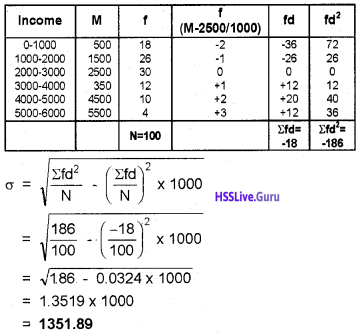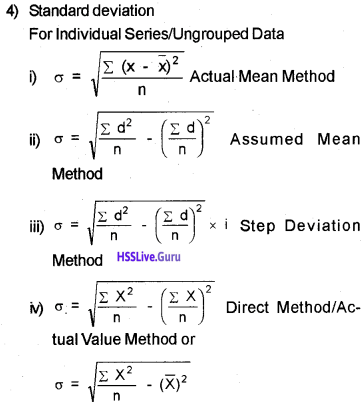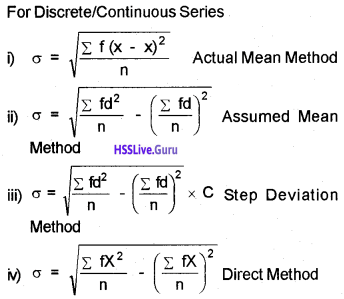# Plus One Economics Chapter Wise Questions and Answers Chapter 6 Measures of Dispersion

Students can Download Chapter 6 Measures of Dispersion Questions and Answers, Plus One Economics Chapter Wise Questions and Answers helps you to revise the complete Kerala State Syllabus and score more marks in your examinations

## Kerala Plus One Economics Chapter Wise Questions and Answers Chapter 6 Measures of Dispersion

### Plus One Economics Measures of Dispersion One Mark Questions and Answers

Question 1.
Find out the measure of dispersion from the following.
(i) Range
(ii) Mean deviation
(iii) Standard deviation
(iv) All the above
(iv) All the above

Question 2.
Variance is equal to:
(i) SD
(ii) SD²
(iii) √SD
(iv) SD/2
(ii) SD²

Question 3.
Lorenz curve is used to measure
(i) Literacy rate
(ii) Unemployment
(iii) Inequality
(iv) None of these
(iii) InequalityQuestion 4.
Which is the graphical method of determining dispersion?
(i) Range
(ii) Q.D
(iii) S.D
(iv) Lorenz curve
(iv) Lorenz curve

Question 5.
Which among the following is not an absolute measure of dispersion?
(i) Range
(ii) Co-efficient of variation
(iii) Mean deviation
(iv) Standard deviation
(ii) Co-efficient of variation

Question 6.
Which of the following is the most widely used measure of dispersion?
(i) Range
(ii) Standard deviation
(iii) Mean deviation
(iv) Quartile deviation
(ii) Standard deviation

Question 7.
Which of the following is useful in comparing the variability of two or more distributions?
(i) Lorenz Curve
(ii) Standard deviation
(iii) Mean deviation
(iv) Quartile deviation
(iii) Mean deviation

Question 8.
Which of the following is not based on all the values?
(i) Mean deviation from mean
(ii) Mean deviation from median.
(iii) Range
(iv) Standard deviation
(iii) Range

Question 9.
Mean deviation
(i) is least when calculated from median and higher when calculated from mean.
(ii) is least when calculated from mean and higher when calculated from median.
(iii) same when calculated from median and mean.
(iv) cannot be calculated either from median or mean.
(i) is least when calculated from median and higher when calculated from mean.Question 10.
Mention the purpose for which Lorenz Curve is drawn.
Lorenz curve is drawn for the presentation of inequality (or dispersion)

### Plus One Economics Measures of Dispersion Two Mark Questions and Answers

Question 1.
Which measure of dispersion is the best and how?
Standard deviation is the best measure of dispersion. Because it possesses most of the characteristics of an ideal measure of dispersion.

Question 2.
Range is not based on all the values. Do you agree? Substantiate.
Yes, I agree with the statement that range is not based on all the values. It is simply the difference between the largest (L) and the smallest value (S) in a distribution. Therefore, range cannot be considered as a good measure of dispersion.

Question 3.
Give two limitations of range.
Given below two limitations of range.

1. range is unduly affected by extreme values
2. range is not based on all values.

Question 4.
Differentiate between range and interquartile range.
Range is the difference between the value of extreme while interquartile range is the difference between quarter extreme values of the series.

Question 5.
Some measures of dispersion depend upon the spread of values whereas some calculate the variation of values from a central value. Do you agree?
Yes, I agree with this statement. Range and quartile deviation measure the dispersion by calculating the spread within which the values lie. That is they de-pend.on the spread of values. On the other hand, mean deviation and standard deviation calculate the variation of value from a central value.Question 6.
Write the merits and demerits of mean deviation.
1. Merits

• It is based on all observations
• It is less affected by extreme items
• It is easy to calculate & simple to understand

2. Demerits

• It ignores + signs
• It is difficult to compute when
• or median comes infraction.

### Plus One Economics Measures of Dispersion Three Mark Questions and Answers

Question 1.
Calculate coefficient of standard deviation from the following information SD = 22.4, Mean = 159
Coefficient of standard deviation = $$\frac{\mathrm{SD}}{\bar{x}} \times 100$$
$$=\frac{22.4}{159} \times 100=0.14 \times 100=14$$

Question 2.
Following are certain measures of dispersion. Classify them into absolute measures and relative measures.
{Range, Coefficient of variation, Mean Deviation, Coefficient of Quartile Deviation, coefficient of Range, Standard deviation}Question 3.

1. range, quartile deviation, mode, standard deviation
3. Range, Mean Deviation, coefficient of variation, Standard deviation.

1. Mode. Others are methods of studying measures of dispersion.
2. Correlation. Others are different expressions of dispersion.
3. Coefficient of variation. Others are absolute measures of dispersion.

Question 4.
Calculate the coefficient of variation, if,
Number of Boys = 72
Average Height = 68
The variance of distribution = 9Question 5.
Point out features of good measure of dispersion.
The features of a good measure of dispersion are pointed out below:

• It should be easy to understand
• It should be simple to calculate
• It should be rigidly defined
• It should be based on all observations
• It should not be affected by extreme valuesQuestion 6.
The size of landholdings of 5 persons are given below:

 Individuals Land in acres A 1.5 B 3.0 C 0.5 D 6.0 E 2.0

1. Analyse the data by measuring the dispersion using the easiest method.
2. What are the drawbacks of that method?

1. Range is the simplest method of studying dispersion.
Range = L – S
where L = Largest item
S = Smallest item
Range = 6 – 0.5 = 5.5 acres
2.

• It is not based on each and every item of the distribution.
• It is subject to fluctuations from sample to sample.
• It cannot be computed in case of open-end classes.

Question 7.
If the sum of 10 values is 100 and the sum of their square is 1090, find the coefficient of variation.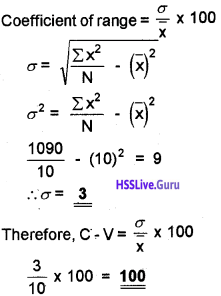### Plus One Economics Measures of Dispersion Four Mark Questions and Answers

Question 1.
In a town, 25% of the persons earned more than Rs 45,000 whereas 75% earned more than 18,000. Calculate the absolute and relative values of dispersion.
We get the following information from the above.
Q1 = Rs. 18000 and Q3 = Rs. 45000
Absolute measure of dispersion (quartile deviation)
= Q3 – Q1/2 = 45000 – 18000/2
= Rs. 13500
Relative measure of dispersion (coefficient of Q. D)
= Q3-Q1/Q3 + Q1
= 45000 -18000 / 45000 + 18000
= 27000/63000
= 3/7
= 0.43

Question 2.
What do you mean by dispersion? Name the important methods of measuring dispersion.
Dispersion is the extent to which values in a distribution differ from the average of the distribution.
To quantify the extent of the variation, there are certain measures namely:

1. Range
2. Quartile Deviation
3. Mean Deviation
4. Standard Deviation
5. Lorenz curve

Question 3.
Mention the merits of standard deviation.
Merits of standard deviation are:

1. It is rigidly defined
2. It is based on all observations
3. It takes algebraic signs in consideration
4. It serves the basis of other measures like correlation.

Question 4.
The number of telephone calls received at an exchange in 245 successive one-minute intervals are shown in the following frequency distribution. Compute the mean deviation about the median.

 No. of calls Frequency 0 14 1 21 2 25 3 43 4 51 5 40 6 39 7 12Question 5.
The coefficient of variation of two series are 58% and 69% and their standard deviation are 21.2 and 15.6. Calculate their mean.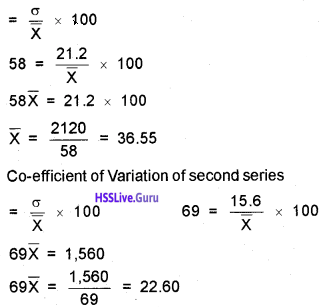Question 6.
Calculate the standard deviation.

 Marks Frequency 20 – 40 3 40-80 6 80-100 20 100-120 12 120-140 9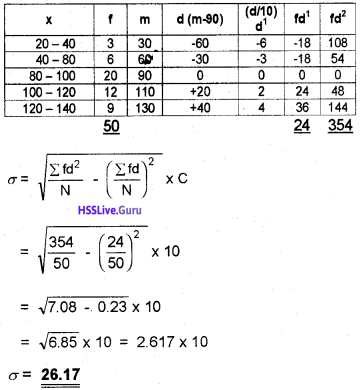### Plus One Economics Measures of Dispersion Five Mark Questions and Answers

Question 1.
Match the following.

 A B Measures of dispersion Karl Pearson Standard deviation SD2 Graphical method SD/mean x 100 Variance Average of the second order Quartile deviation Semi-interquartile range Coefficient of variation Lorenz curve

 A B Measures of dispersion Average of the second order Standard deviation Karl Pearson Graphical method Lorenz curve Variance SD2 Quartile deviation Semi-inter quartile range Coefficient of variation SD/mean x 100

### Plus One Economics Measures of Dispersion Eight Mark Questions and Answers

Question 1.
What do you mean by Lorenz curve? Give the steps required in the Construction of the Lorenz Curve.
The measures of dispersion discussed so far give a numerical value of dispersion. A graphical measure called Lorenz Curve is available for estimating dispersion. In constructing a Lorenz curve, the following steps are involved.

1. Calculate class mid-points and find cumulative totals
2. Calculate cumulative frequencies
3. Express the grand totals and convert the cumulative totals in these columns into percentages,
4. Now, on the graph paper, take the cumulative percentages of the variable on Y-axis and cumulative percentages of frequencies on X-axis. Thus each axis will have values from ‘0’to ‘100’.
5. Draw a line joining Co-ordinate (0, 0) with (100,100). This is called the line of equal distribution
6. Plot the cumulative percentages of the variable with corresponding cumulative percentages of frequency.
7. Join these points to get the curve OAC.Question 2.
Calculate

1. standard deviation and
2. mean deviation

from the following data.
X = 7, 9, 18, 11, 10, 8, 17, 13, 11, 16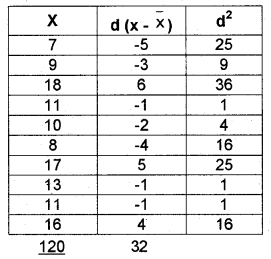1. Standard deviation
$$s=\sqrt{\frac{\sum d^{2}}{N}}$$
$$=\sqrt{\frac{134}{10}}=3.66$$

2. Median deviation
$$M D=\frac{\Sigma|d|}{N}=\frac{32}{10}=3.2$$

Question 3.
The following table gives the distribution of income of 100 families in a village. Calculate standard deviation.

 Income No. of Families 0-1000 18 1000-2000 26 2000-3000 30 3000 – 4000 12 4000 – 5000 10 5000 – 6000 4